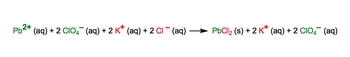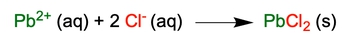Start typing, then use the up and down arrows to select an option from the list.## General Chemistry

Learn the toughest concepts covered in Chemistry with step-by-step video tutorials and practice problems by world-class tutors

6. Chemical Quantities & Aqueous Reactions

# Complete Ionic Equations

Complete Ionic Equations show aqueous compounds as fully dissociated ions.

Complete Ionic Equations
1
concept

## Complete Ionic Equations47s
Play a video:
complete. Ionic equations show acquis compounds as associated ions. Now, remember, in a molecular equation, we have our acquis compounds. But we also possess solids, liquids and gasses. When it comes to solid liquid and gas is, they themselves never break up into ions. It's on Lee the acquis compounds. Remember, we use the Saudi ability rules to determine if something is a kiss or not. Now, when it comes to the complete ionic equation, we're gonna say it comes from the molecular equation. And because of that, you're gonna have to remember to distribute the coefficient off each compound to determine the correct number of ions. So as we go into going from the molecular equation toe are complete ionic equations. We'll see what that entails.

The complete ionic equation shows all the aqueous compounds broken up into ions.2
example

## Complete Ionic Equations Example 12m
Play a video:
in this example. Question, it says convert the following molecular equation into a complete ionic equation. So here we have three moles of calcium bromide, acquis reacting with two moles of lithium phosphate, acquis producing six moles, a little lithium bromide as an acquis compound plus one mole of calcium phosphate. Solid. All right, so remember, we can Onley break up acquis compounds so only these three compounds will break up. If you are solid a liquid or gas, you will not break up in the complete ionic equation. Also, remember that the coefficient gets distributed to each one of these compounds. Not what exactly does that mean? Well, here that means that this three is gonna get distributed to the number of calcium and the number of bro means so it gets distributed to see, um c a N b r. When this breaks up into its ions, we know that we're gonna have calcium ion, a quiz plus bromide ion a quiz. Then we distribute the coefficient, so that's gonna give us three calcium ions and then this is three times to six bromide ions. Plus, also, remember that when we have an eye on, it's an aqueous phase within a solution. This too is gonna get distributed to hear and to hear. So we know that's going to give us six lithium ions because it is two times three plus two phosphate ions. Remember, phosphate is a poly atomic ion produces six gets distributed to lithium bromide. So six lithium plus one ions plus six bromide ions. This is a solid. So it stays together, does not break up in tow ions. So plus one so one not 61 calcium phosphate solid. So this year represents our complete ionic equation. Remember to break up on Lee Equus compounds and remember to distribute the ions to each one of distribute number of coefficients to each one of those ions that you form.
3
concept

## Complete Ionic Equations51s
Play a video:
now a net ionic equation shows the ions participating in the chemical reaction by removing what we call the spectator ions. These spectator ions represent compounds that are both reactant and products. So they're found on both sides of an equation, but they're not part of ah, net Ionic equation. Now, when it comes to the net Ionic equation, we're gonna say it comes from the complete Ionic equation. So remember we started out with our molecular equation. From there, we're able to determine our complete ionic equation. And then from our complete ionic equation, we now are looking at our Net Ionic equation. Mhm. So this is the path you have to take to get to our Net ionic equation. Now that we know how they're connected, let's move on to the next video and take a look at an example Question

Net Ionic Equation shows only the ions participating in the chemical reaction, without the spectator ions.4
example

## Complete Ionic Equations Example 24m
Play a video:
based on the given reactant provide both the molecular equation and the complete ionic equation. So here we have ammonium sulfate react with calcium chloride. So for steps zero, we're just going to follow steps 1 to 4 that we've learned in the past to first give the molecular equation. Now, remember, when it comes to these steps were first going to break up each of these compounds into their ionic forms. So ammonium sulfate breaks up into the ammonium ion and the sulfate ion. Don't worry about the fact that we have to Ammonium is here. We'll discuss that later on as we're going into the complete Ionic equation, and then calcium chloride breaks up into calcium ion and chloride ion. Now, remember, we're gonna swap Ionic Partners because opposite charges attract this. Plus one charge is attracted to this minus one charge. So when they connect together, remember when the numbers are the same, they just simply cancel out. So here, that will give me an H four c l plus, Then we're gonna have here calcium ion and sulfate ion. The numbers and the charges again are the same. So they're going to cancel out. Give me calcium sulfate. At this point, we have to remember our saw You bility rules to see if we created Ah, solid liquid or gas. Otherwise, no reaction has occurred. So remember, based on our side ability rules, anything connected to the ammonium ion will be soluble. So this is a quiz, and then we have calcium sulfate based on the rules that we learned about calcium sulfate, remember, it's gonna form a precipitate if sulfate ion is connected to CBS. Remember, CBS stands for calcium, barium or strontium. Since sulfate here is connected to calcium, it's gonna form a precipitate. Since we made a solid, a reaction has occurred. Next we just have to balance out this molecular equation. We have to. Ammonium is here and to chorines here, So I have to put a two right here. The sulfate There's just one sulfate and one sulfate, one calcium and one calcium. So here we have coefficients of one for the other compounds. Now we're going to go to step five where we break up on Lee the acquis compounds into the respective ions. So everyone breaks up into ions except for calcium sulfate, which is a solid. So here remember, the coefficient gets distributed to each of these ions we're gonna have here one ammonium ion, acquis. But remember this little to here, so there's actually two ammonium ions. Plus, we're gonna have sulfate ion acquis plus one calcium ion. Acquis. Plus, we have a two here to chloride ions. A quiz gives us The two gets distributed to each one of these ions here, so we're gonna have to ammonium ions. Acquis plus two chloride ions. A quiz plus one. Calcium sulfate solid. Here. We're gonna cancel out the spectator ions from the complete Ionic equation in orderto isolate the Net Ionic equation. At this point, what we have is the complete ionic equation for the net. Ionic, Let's remove the spectator ions. Remember, spectator ions are the compounds that exists is both reactant and products at the same time. So here, ammonium ion here matches up with ammonium ion here. So those will not be part of the net. Ionic Also we have here to chlorides and to chlorides. They also will not be part of the net Ionic. That means all that's left at the end. When we bring things down, will be s 04 to minus acquis, plus calcium. Ion acquis gives me calcium sulfate solid. So this year represents my Net Ionic equation. So just remember a lot of what we learned in terms of obtaining the Net Ionic equation has the first deal with determining the molecular equation. From there, we have to look at the complete Ionic equation and by removing the spectator ions, that's how we're able to isolate our Net Ionic equation at the very end.
5
Problem

Provide the net ionic equation that occurs when the following aqueous compounds are mixed together:

Copper (II) Bromide and Lithium Hydroxide